GeeksforGeeks App
Open AppBrowser
Continue

# Cleaning data with dropna in Pyspark

While dealing with a big size Dataframe which consists of many rows and columns they also consist of many NULL or None values at some row or column, or some of the rows are totally NULL or None. So in this case, if we apply an operation on the same Dataframe that contains many NULL or None values then we will not get the correct or desired output from that Dataframe. For getting the correct output from the Dataframe we have to clean it, which means we have to make Dataframe free of NULL or None values.

So in this article, we will learn how to clean the Dataframe. For cleaning the Dataframe we are using dropna() function. This function is used to drop the NULL values from the Dataframe on the basis of a given parameter.

Syntax: df.dropna(how=”any”, thresh=None, subset=None)

where, df is the Dataframe

Parameter:

• how: This parameter is used to determine if the row or column has to remove or not.
• ‘any’ – If any of the value in Dataframe is NULL then drop that row or column.
• ‘all’ – If all the values of particular row or column is NULL then drop.
• thresh: If non NULL values of particular row or column is less than thresh value then drop that row or column.
• subset: If the given subset column contains any of the null value then dop that row or column.

To drop the null values using the dropna method, first, we will create a Pyspark dataframe and then apply this.

## Python

 `# importing necessary libraries``from` `pyspark.sql ``import` `SparkSession` `# function to create new SparkSession``def` `create_session():``    ``spk ``=` `SparkSession.builder \``        ``.master(``"local"``) \``        ``.appName(``"Employee_detail.com"``) \``        ``.getOrCreate()``    ``return` `spk`  `def` `create_df(spark, data, schema):``    ``df1 ``=` `spark.createDataFrame(data, schema)``    ``return` `df1`  `if` `__name__ ``=``=` `"__main__"``:` `    ``# calling function to create SparkSession``    ``spark ``=` `create_session()` `    ``input_data ``=` `[(``1``, ``"Shivansh"``, ``"Data Scientist"``, ``"Noida"``),``                  ``(``2``, ``None``, ``"Software Developer"``, ``None``),``                  ``(``3``, ``"Swati"``, ``"Data Analyst"``, ``"Hyderabad"``),``                  ``(``4``, ``None``, ``None``, ``"Noida"``),``                  ``(``5``, ``"Arpit"``, ``"Android Developer"``, ``"Banglore"``),``                  ``(``6``, ``"Ritik"``, ``None``, ``None``),``                  ``(``None``, ``None``, ``None``, ``None``)]``    ``schema ``=` `[``"Id"``, ``"Name"``, ``"Job Profile"``, ``"City"``]` `    ``# calling function to create dataframe``    ``df ``=` `create_df(spark, input_data, schema)``    ``df.show()`

Output: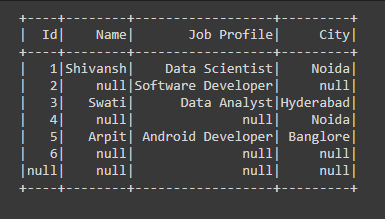Example 1: Cleaning data with dropna using any parameter in PySpark.

In the below code we have passed the how=”any” parameter in the dropna() function which means that if there are any row or column which has any of the Null values then we are dropping that row or column from the Dataframe.

## Python

 `# if any row having any Null``# value we are dropping that``# rows``df ``=` `df.dropna(how``=``"any"``)``df.show()`

Output: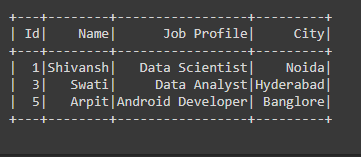Example 2: Cleaning data with dropna using all parameters in PySpark.

In the below code, we have passed the how=”all” parameter in the dropna() function which means that if there are all row or column which has all the Null values then we are dropping that particular row or column from the Dataframe.

## Python

 `# if any row having all Null``#  values we are dropping that``# rows.``df ``=` `df.dropna(how``=``"all"``)``df.show()`

Output: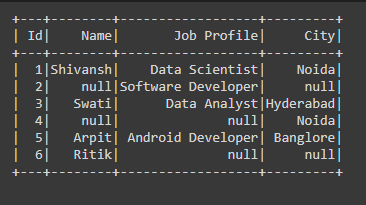Example 3: Cleaning data with dropna using thresh parameter in PySpark.

In the below code, we have passed the thresh=2 parameter in the dropna() function which means that if there are any rows or columns which is having fewer than non-NULL values than thresh values then we are dropping that row or column from the Dataframe.

## Python

 `# if thresh value is not``# satisfied then dropping``# that row``df ``=` `df.dropna(thresh``=``2``)``df.show()`

Output: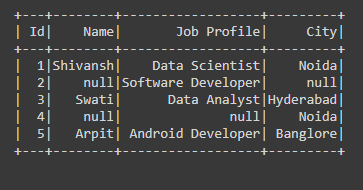Example 4: Cleaning data with dropna using subset parameter in PySpark.

In the below code, we have passed the subset=’City’ parameter in the dropna() function which is the column name in respective of City column if any of the NULL value present in that column then we are dropping that row from the Dataframe.

## Python

 `# if the subset column any value``# is NULL then dropping that row``df ``=` `df.dropna(subset``=``"City"``)``df.show()`

Output: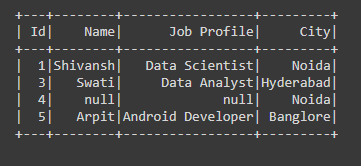Example 5: Cleaning data with dropna using thresh and subset parameter in PySpark.

In the below code, we have passed (thresh=2, subset=(“Id”,”Name”,”City”)) parameter in the dropna() function, so the NULL values will drop when the thresh=2 and subset=(“Id”,”Name”,”City”) these both conditions will be satisfied means among these three columns dropna function checks whether thresh=2 is also satisfying or not, if satisfied then drop that particular row or column.

## Python

 `# if thresh value is satisfied with subset``# column then dropping that row``df ``=` `df.dropna(thresh``=``2``,subset``=``(``"Id"``,``"Name"``,``"City"``))``df.show()`

Output: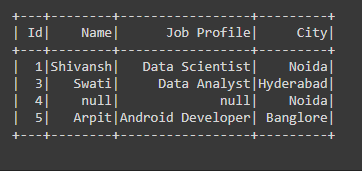My Personal Notes arrow_drop_up